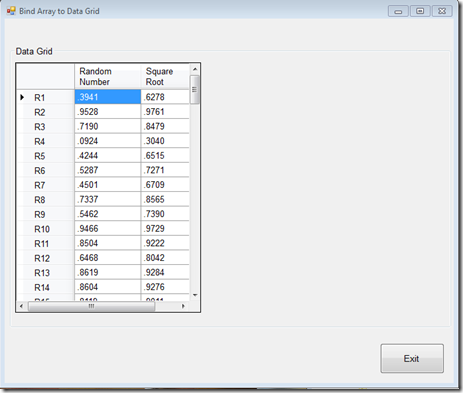Geeks With Blogs
Jim Lahman's Blog Fortitude|Endurance|Faith|Teamwork

Here is a technique to bind a List (of objects) to a DataGridView so that the public Properties appear as columns.In this example, the columns are binded to the individual public property of the object.  The column Random Number is binded to the public property RandomNumber while the column Square Root to the public property  SqRt

Create a class that contains the public properties that appear as columns in the dataGridView

`   1:  using System;`
`   2:  using System.Collections.Generic;`
`   3:  using System.Linq;`
`   4:  using System.Text;`
`   5:   `
`   6:  namespace TestBindArrayToGrid`
`   7:  {`
`   8:      public class RandomNbr : System.Object`
`   9:      {`
`  10:          private Double m_RandomNbr = 0.0;`
`  11:          private Double m_SqRt = 0.0;`
`  12:   `
`  13:          private Random r;`
`  14:   `
`  15:          /* Each array list is a new object but we do not want to reseed the random number algorithm.  So`
`  16:           * we create this constructor to pass in the random number into the new object`
`  17:           */`
`  18:          public RandomNbr(Double RandomNbr)`
`  19:          {`
`  20:              m_RandomNbr = RandomNbr;`
`  21:              m_SqRt = Math.Sqrt(RandomNbr);`
`  22:          }`
`  23:   `
`  24:          /* This constructor generates the initial random number */`
`  25:          public RandomNbr()`
`  26:          {`
`  27:              m_RandomNbr = GenerateRandomNumber();`
`  28:              m_SqRt = Math.Sqrt(m_RandomNbr);`
`  29:          }`
`  30:   `
`  31:          /* This public property is binded to the Random Number column */`
`  32:          public Double RandomNumber  `
`  33:          {`
`  34:              get { return this.m_RandomNbr; }`
`  35:              set { m_RandomNbr = value; }`
`  36:          }`
`  37:   `
`  38:          /* This public property in binded to the Square Root column */`
`  39:          public Double SqRt`
`  40:          {`
`  41:              get { return this.m_SqRt; }`
`  42:              set { m_SqRt = value; }`
`  43:          }`
`  44:   `
`  45:          /* This seeds the random number algorithm */`
`  46:          private double GenerateRandomNumber()`
`  47:          {`
`  48:              TimeSpan ts = DateTime.Now.Subtract(new DateTime(2011,01,01));`
`  49:              var Seconds = ts.TotalSeconds;`
`  50:   `
`  51:              r = new Random((int) Seconds);`
`  52:              return r.NextDouble();`
`  53:          }`
`  54:   `
`  55:          /* Return the next random number as generated by the algorithm */`
`  56:          public Double GetNextRandom()`
`  57:          {`
`  58:              return r.NextDouble();`
`  59:          }`
`  60:   `
`  61:          /* For those cases when a random number and square root are to be returned. */`
`  62:          public void GetNextRandom(ref double _RandonNbr, ref double _SqRt)`
`  63:          {`
`  64:              _RandonNbr = m_RandomNbr = r.NextDouble();`
`  65:              _SqRt = m_SqRt = Math.Sqrt(m_RandomNbr);`
`  66:          }`
`  67:   `
`  68:      }`
`  69:  }`

And here is the technique to bind the List<T> objects to the DataGridView

`   1:  using System;`
`   2:  using System.Collections.Generic;`
`   3:  using System.ComponentModel;`
`   4:  using System.Data;`
`   5:  using System.Drawing;`
`   6:  using System.Linq;`
`   7:  using System.Text;`
`   8:  using System.Windows.Forms;`
`   9:   `
`  10:  namespace TestBindArrayToGrid`
`  11:  {`
`  12:      public partial class frmBindArrayToGrid : Form`
`  13:      {`
`  14:          List<RandomNbr> lstRandom = new List<RandomNbr>();  // List<T>`
`  15:          private RandomNbr r = new RandomNbr();      // This seeds the random number generator`
`  16:   `
`  17:          public frmBindArrayToGrid()`
`  18:          {`
`  19:              InitializeComponent();`
`  20:          }`
`  21:   `
`  22:          private void cmdExit_Click(object sender, EventArgs e)`
`  23:          {`
`  24:              Application.Exit();`
`  25:          }`
`  26:   `
`  27:          private void frmBindArrayToGrid_FormLoad(object sender, EventArgs e)`
`  28:          {`
`  29:              GenerateRandomList();`
`  30:              BindArrayListToGrid();`
`  31:          }`
`  32:   `
`  33:          /* This binds each list object to the datagridview */`
`  34:          private void BindArrayListToGrid()`
`  35:          {`
`  36:              dgRandom.Columns.Clear();`
`  37:   `
`  38:              // Create new column for randon number`
`  39:              DataGridViewTextBoxColumn csRandom = new DataGridViewTextBoxColumn();`
`  40:              `
`  41:              csRandom.DataPropertyName = "RandomNumber";  // Public property name (as defined in the object)`
`  42:              csRandom.HeaderText = "Random Number";      // Header name`
`  43:              csRandom.DefaultCellStyle.Format = "#.#000";    // Format `
`  44:              dgRandom.Columns.Add(csRandom);`
`  45:   `
`  46:              // Create new column for square root`
`  47:               DataGridViewTextBoxColumn csSqRt = new DataGridViewTextBoxColumn();`
`  48:   `
`  49:              csSqRt.DataPropertyName = "SqRt";  // Public property name (as defined in the object)`
`  50:              csSqRt.HeaderText = "Square Root";      // Header name`
`  51:              csSqRt.DefaultCellStyle.Format = "#.#000";    // Format `
`  52:              dgRandom.Columns.Add(csSqRt);            `
`  53:   `
`  54:              dgRandom.DataSource = lstRandom;        // Binding is to the list of random numbers`
`  55:              /* Create the row headers */`
`  56:              int rowNumber = 1;`
`  57:              foreach (DataGridViewRow row in dgRandom.Rows)`
`  58:              {`
`  59:                  row.HeaderCell.Value = "R" + rowNumber;`
`  60:                  rowNumber++;`
`  61:              }`
`  62:              dgRandom.AutoResizeRowHeadersWidth(DataGridViewRowHeadersWidthSizeMode.AutoSizeToAllHeaders);`
`  63:   `
`  64:              dgRandom.Show();`
`  65:          }`
`  66:   `
`  67:          private void GenerateRandomList()`
`  68:          {`
`  69:              /* For each new list <object>, retrieve the next random number from the algorithm */`
`  70:              lstRandom.Add(new RandomNbr(r.GetNextRandom()));  `
`  71:              for (int i = 0; i < 100; i++)`
`  72:              {`
`  73:                  lstRandom.Add(new RandomNbr(r.GetNextRandom()));`
`  74:              }`
`  75:          }`
`  76:      }`
`  77:  }`